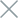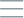• Home
• Tags
• Series
•# Multi-Target Search Algorithm in PHP: Exploring Concepts and Examples

Multi-target search is an important algorithm in programming that allows searching for multiple values in a data set simultaneously. This algorithm addresses practical issues in programming, such as finding elements that satisfy a condition within a list or an array.

## Algorithm Operation

The multi-target search algorithm generally operates similarly to the algorithm for searching a single target. However, instead of stopping after finding a single target, it continues searching to find all targets that satisfy the condition. The general procedure of the algorithm is as follows:

1. Iterate through each element in the data set.
2. Check the condition for each element. If the element satisfies the condition, add it to the result list.
3. Continue iterating through other elements to find other targets that satisfy the condition.
4. Return the result list containing all targets that satisfy the condition.

• The multi-target search algorithm is versatile and applicable in various situations.
• It saves time and effort compared to performing separate loops to find each target.

• The algorithm's performance can be affected when dealing with large data sets and a high number of targets that satisfy the condition.
• It may lead to memory inefficiencies when storing a large result list with many targets.

## Example and Explanation

Let's assume we have a list of integers and we want to find all numbers that are multiples of 3 in this list. Below is an example of using the multi-target search algorithm in PHP:

``````function findMultiplesOfThree(\$numbers) {
\$result = array();

foreach (\$numbers as \$number) {
if (\$number % 3 === 0) {
\$result[] = \$number; // Add the satisfying number to the result list
}
}

return \$result;
}

\$numbers = array(9, 4, 15, 7, 12, 6);
\$multiplesOfThree = findMultiplesOfThree(\$numbers);

echo "Numbers that are multiples of 3 in the list are: ";
foreach (\$multiplesOfThree as \$number) {
echo \$number . " ";
}
``````

In this example, the `findMultiplesOfThree` function iterates through each number in the list. If a number is divisible by 3 (has a remainder of 0 when divided by 3), it is added to the result list. Ultimately, the result list will contain the numbers 9, 15, and 12, which will be printed to the screen.

Therefore, the multi-target search algorithm in PHP helps us easily find and process all targets that satisfy a given condition within a data set.